Section A16.2 example 1 SECTION A16.2 EXAMPLE 1
For the monocoque cylinderical pressure vessel shown, determine the
• (a) hoop stress
• (b) axial stress
• (c) maximun shear stress
If the pressure was increased to 20 psi, what should be the thickness of the skin to maintain the same level of hoop stress as found in part (a)?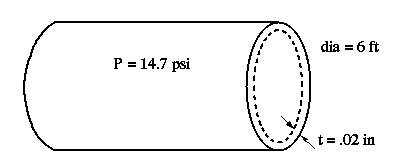The pressure shown is the gauge pressure.

EQUATIONS USED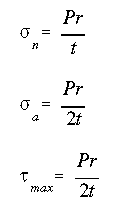SOLUTION

(a) The hoop stress is given as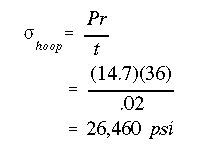(b) The axial stress is found by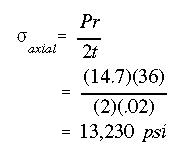(c) The maximum shear stress is determined asIf the pressure was increased to 20 psi, the new skin thickness to maintain the same level of hoop stress would be found as follows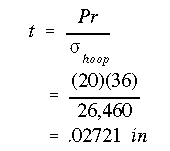Therefore, it takes 36% more skin thickness to carry 36% more pressure for the same level of stress in skin.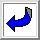To Section A16.2